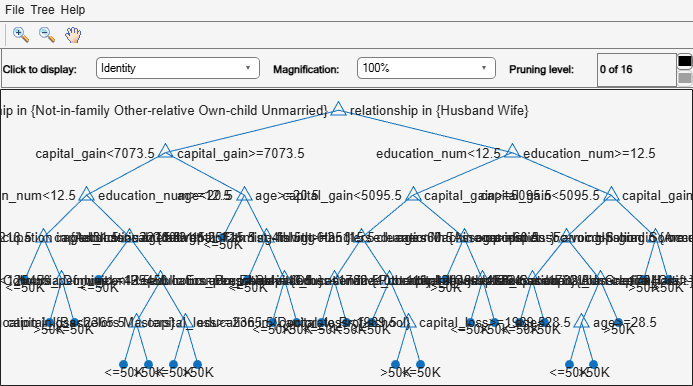# nodeVariableRange

Retrieve variable range of decision tree node

## Syntax

``varRange = nodeVariableRange(tree,nodeID)``
``varRange = nodeVariableRange(tree,nodeID,OmitUnusedVariables=omitUnusedVars)``

## Description

example

````varRange = nodeVariableRange(tree,nodeID)` returns the range of predictor variables `varRange` at the `tree` node specified by `nodeID`.```
````varRange = nodeVariableRange(tree,nodeID,OmitUnusedVariables=omitUnusedVars)` also specifies whether to omit the unused predictor variables from the returned `varRange`.```

## Examples

collapse all

Create a decision tree for classification, and retrieve the range of variables at a specified node of the decision tree.

Load the `census1994` data set. The table `adultdata` contains six numeric and eight categorical variables.

`load census1994`

Train a classification tree based on the features contained in `adultdata` and the class labels in `adultdata.salary`. Limit the number of splits in the tree by specifying the name-value argument `MaxNumSplits`.

`tree = fitctree(adultdata,"salary",MaxNumSplits=31)`
```tree = ClassificationTree PredictorNames: {1x14 cell} ResponseName: 'salary' CategoricalPredictors: [2 4 6 7 8 9 10 14] ClassNames: [<=50K >50K] ScoreTransform: 'none' NumObservations: 32561 Properties, Methods ```

`tree` is a trained `ClassificationTree` model for classification.

View the graphical display of the trained classification tree.

`view(tree,Mode="graph")`Retrieve the range of predictor variables at node 10.

`varRange = nodeVariableRange(tree,10)`
```varRange = struct with fields: age: [-Inf 20.5000] relationship: [Not-in-family Other-relative Own-child Unmarried] capital_gain: [7.0735e+03 Inf] ```

## Input Arguments

collapse all

Decision tree model, specified as one of the following:

Node in the decision tree, specified as a positive integer scalar. `nodeID` must be less than or equal to the number of nodes in the decision tree.

Data Types: `single` | `double`

Indicator to omit unused predictor variables from `varRange`, specified as a numeric or logical `1` (`true`) or `0` (`false`).

## Output Arguments

collapse all

Variable range at the decision tree node, returned as a structure. If a predictor variable is numeric, the corresponding field of `varRange` is a 1-by-2 numeric vector containing the lower and upper bounds. If a predictor variable is categorical, the corresponding field of `varRange` is a categorical array containing the categories subgroup.

## Version History

Introduced in R2020a